Transformations: Rotations MathBitsNotebook.com Terms of Use   Contact Person: Donna RobertsA rotation turns a figure about a fixed point called the center of rotation.
An object and its rotation are the same size and shape,
but the figures may be facing in different directions.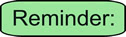Rotations are TURNS!!

Rotations can be seen in a variety of situations:
 The Earth Windmills Pinwheel The Earth experiences one complete rotation on its axis every 24 hours.The blades on windmills convert the energy of wind into rotational energy.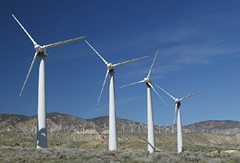A children's toy that rotates when blown.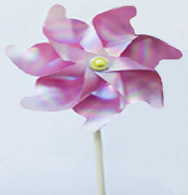Amusement Park Swing Ferris Wheel Merry-Go-Round Amusement park rides, such as the swing, allow you to become part of a rotation.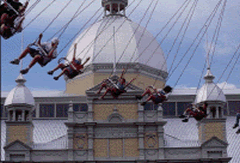Ferris wheels rotate about a center hub. (Yes, the seats tilt to prevent falling.)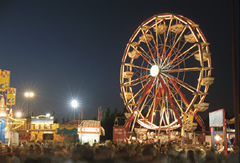On the merry-go-round, riders become part of the rotation about the center of the ride.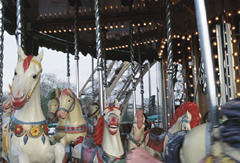A rotation is a transformation that turns a figure about a fixed point called the center of rotation. • The original object is called the pre-image, and the rotation is called the image. • The image is usually labeled using a prime symbol, such as A'B'C'. • An object and its rotation are the same shape and size, but the figures may be turned in different directions. A rigid transformation. • Rotations may be clockwise or counterclockwise. • Naming (lettering) a figure in a translation occurs in the same direction in both figures (such as both clockwise or both counterclockwise).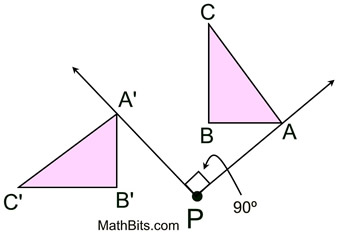The triangle is rotated about P. This is a rotation of 90º counterclockwise. The letters used to label the triangle have not been rotated.

 Properties preserved under a rotation from the pre-image to the image: 1. distance (lengths of segments remain the same) 2. angle measures (remain the same) 3. parallelism (parallel lines remain parallel) 4. collinearity (points remain on the same lines) 5. midpoints (midpoints remain the same in each figure) 6. orientation (lettering order remains the same)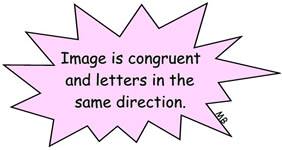The preserved properties of a transformation are referred to as being "invariant" in that they do not vary (change) during the transformation.Rotations in the coordinate plane:

It is true that rotations may occur in a clockwise (CW) or counterclockwise (CCW) direction.
BUT ...

 NOTE: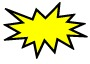It is convention that rotations in the coordinate plane (when graphing), are counterclockwise, unless otherwise stated. A positive angle of rotation turns the figure counterclockwise, and a negative angle of rotation turns the figure in a clockwise direction.

 The convention of rotations being counterclockwise on a coordinate grid is in keeping with the unit circle. (The unit circle is a widely used circle in higher mathematics. It has a radius length of 1 unit.) Notice that the degree movement on a unit circle goes in a counterclockwise direction, the same direction as the numbering of the quadrants: I, II, III, IV. Keep this picture in mind when working with rotations on a coordinate grid.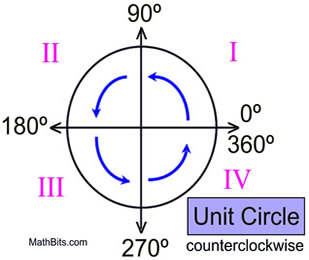When working with rotations, it will be helpful to recognize angles of certain sizes.
Some popular angles are:
 • 30º (one third of a right angle) • 45º (half of a right angle) • 90º (a right angle) • 180º (2 right angles, straight angle) • 270º (3 right angles) • 360º (4 right angles, a full circle)

Most (but not all) rotations will have their "center of rotation" located at the origin (0,0).
The center of rotation will be indicated in the problem (or in the notation).

Counterclockwise rotations centered at origin:
 Rotation of 90º: (x,y) becomes (-y,x) Rotation of 180º: (x,y) becomes (-x,-y) Rotation of 270º: (x,y) becomes (y,-x)
It is convention that rotations in the coordinate
plane are counterclockwise, unless otherwise stated.
 Remember: Clockwise: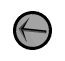Counterclockwise: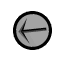Rotation 90º: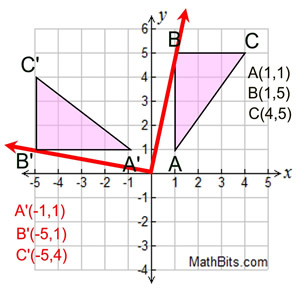Starting with ΔABC, draw the rotation of 90º centered at the origin. (The rotation is counterclockwise.)

To "see" that this is a rotation of 90º, imagine point B attached to the red arrow. The red arrow is then moved 90º (notice the 90º angle formed by the two red arrows). Look at the new position of point B, labeled B'. This same approach can be used for all three vertices.

 Rotation of 90º on coordinate axes. Centered at origin. Counterclockwise. (x, y) → (-y, x)Rotation 180º: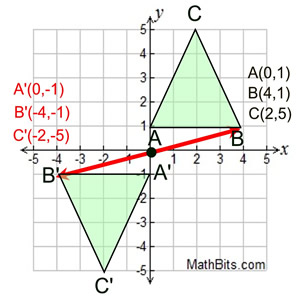Starting with ΔABC, draw the rotation of 180º centered at the origin. (The rotation is counterclockwise.)

As we did in the previous example, imagine point B attached to the red arrow from the center (0,0). The arrow is then moved 180º (which forms a straight line). Notice the new position of B, labeled B'.

 Rotation of 180º on coordinate axes. Centered at origin. Counterclockwise. (x, y) → (-x, -y) (same as a point reflection in origin)Rotation 270º: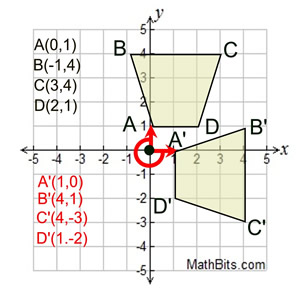Starting with quadrilateral ABCD, draw the rotation of 270º centered at the origin. (The rotation is counterclockwise.)

As we did in the previous examples, imagine point A attached to the red arrow from the center (0,0). The arrow is then moved 270º (counterclockwise). Notice the new position of A, labeled A'. Since A was "on" the axis, A' is also on the axis under a turn of 270º (which is three 90º turns).

 Rotation of 270º on coordinate axes. Centered at origin. Counterclockwise. (x, y) → (y, -x )
The notation for a rotation will be a capital R with a subscript of the location of the center of the rotation (O stands for origin) and the number of counterclockwise degrees for the rotation.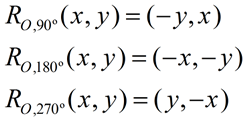The formulas for rotations are universally written as shown above, using a counterclockwise rotation.

While formulas for clockwise rotations can be written, it is more often the case that clockwise rotations are referred to by their counterclockwise counterparts.
90º clockwise = 270º counterclockwise
180º clockwise = 180º counterclockwise
270º clockwise = 90º counterclockwise

 Counterclockwise Rotation Formula Clockwise Rotation 90º (x,y) becomes (-y,x) 270º 180º (x,y) becomes (-x,-y) 180º 270º (x,y) becomes (y,-x) 90º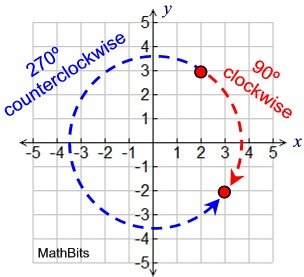Point (2,3) becomes (3,-2)
under a 90º clockwise rotation
or a 270º counterclockwise rotation.
Clockwise rotations are also referred to as "negative" counterclockwise rotations.
A clockwise rotation of 90º is also a counterclockwise rotation of -90º.
A clockwise rotation of 180º is also a counterclockwise rotation of -180º.
A clockwise rotation of 270º is also a counterclockwise rotation of -270º.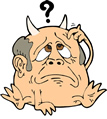HINT: Having problems picturing what rotations will look like? A nice thing about coordinate grid problems is that you can turn the graph paper to see what certain rotations will look like.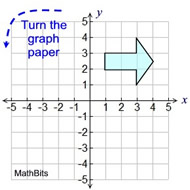To see a 90º counterclockwise rotation, turn the graph paper one turn to the left.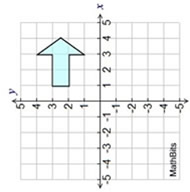What a 90º counterclockwise rotation will look like.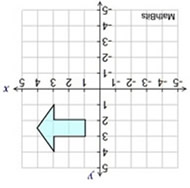Turn left again to see a 180º counterclockwise rotation.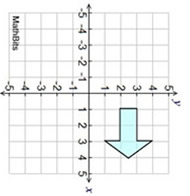Turn left again to see a 270º counterclockwise rotation.

Yes, the scales and the axes are messed up, but your view of the figure is correctly positioned.
Just re-adjust the axes and the scales.
This also works for "clockwise" rotations - just turn the graph paper to the right (instead of left).If the rotation angles are giving you trouble, imagine a unit circle with a movable "bug" on a radial arm from the origin. Swing the "bug" around and look at the angle created by the move, and the position of the "bug".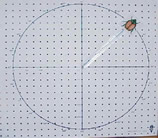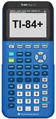For calculator help with rotations click here.